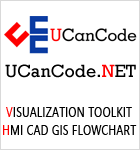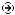YOU CAN CODE!With The Case Of UCanCode.net  Release The Power OF  Visual C++ ! Home |  Products | Purchase |  Support | DownloadsDownload EvaluationPricing & Purchase?E-XD++Visual C++/ MFC ProductsOverviewElectronic Form SolutionVisualization & HMI SolutionPower system HMI SolutionBar code labeling SolutionWorkflow SolutionCoal industry HMI SolutionInstrumentation Gauge SolutionReport Printing SolutionGraphical modeling SolutionGIS mapping solutionVisio graphics solutionIndustrial monitoring SolutionFlowchart and diagramming SolutionOrganization Diagram SolutionGraphic editor Source CodeUML drawing editor Source CodeMap Diagramming SolutionArchitectural Graphic Drawing SolutionRequest EvaluationActiveX COM ProductsOverviewTechnical Support# Get Ready to Unleash the Power of UCanCode .NET

UCanCode Software focuses on general application software development. We provide complete solution for developers. No matter you want to develop a simple database workflow application, or an large flow/diagram based system, our product will provide a complete solution for you. Our product had been used by hundreds of top companies around the world!

"100% source code provided! Free you from not daring to use components because of unable to master the key technology of components!"

 GDI Example: Add and Draw US flag with E-XD++, five star shape

With E-XD++ you can create any diagram quickly and easily, for example, if you want to create a new diagram  like below, E-XD++ will be the best component to build and UML Diagram Drawing Tool:It is tested on Visual Studio 6.0, Visual Studio.net 2013 and Visual Studio.net 2022.

Example codes as below:

{
CFORectShape *pRect = new CFORectShape;
pRect->Create(rc, "");
pRect->UpdateComp();
pRect->Release();

return pRect;
}

{
CFOFiveStarShape *pStar = new CFOFiveStarShape;
pStar->Create(rc, "");
pStar->UpdateComp();
pStar->Release();

return pStar;
}

#define SIN72 0.95106
#define COS72 0.30902
#define SIN144 0.58779
#define COS144 -0.80902
#define STARSIZE 0.67 // scale factor for the size of the stars
void CTestMDIView::GenUSFlag(RECT * pRect)
{
int x0 = pRect->left;
int y0 = pRect->top;
int x1 = pRect->right;
int y1 = pRect->bottom;
int cx = x1 - x0;
int cy = y1 - y0;
int i, j, x, y, xx, yy;
int cxStarBox, cyStarBox;

CRect rc(x0, y0, x1 + 1, y1 + 1);

// Draw the seven red stripes.
for (i = 0; i < 13; i++)
{
if ((i & 1) == 0)
{
rc = CRect(x0,
y0 + (i * cy) / 13,
x1 + 1,
y0 + ((i + 1) * cy) / 13 + 1);
pRectShape->SetBkColor(RGB(255,0,0));
}
}

// Draw blue box.
// Size it so that it covers two fifths of the flag length and
// the top seven stripes vertically.
cxStarBox = (2 * cx) / 5;
cyStarBox = (7 * cy) / 13 + 1;

rc = CRect(x0,
y0,
x0 + cxStarBox,
y0 + cyStarBox);
pRectShape->SetBkColor(RGB(0, 0, 128));

// Shrink the blue box rect a bit to give some border around the stars.
x0 += cxStarBox / 25;
y0 += cyStarBox / 25;
cxStarBox *= 23;
cxStarBox /= 25;
cyStarBox *= 23;
cyStarBox /= 25;
y = y0;
// Now, divide the box into a grid of 11 x 9 squares and place a star
// in every other square, like on a checker board.
for (j = 1; j <= 9; j++)
{
yy = y0 + (j * cyStarBox) / 9;
x = x0;
for (i = 1; i <= 11; i++)
{
xx = x0 + (i * cxStarBox) / 11;
// Determine 'black' or 'white' square status by checking to see
// if the sum of 'i' and 'j' is odd or even.
// This ensures that the square colors of each row are always
// staggered regardless of whether there is an odd or even number
// of squares in each row.
if (((i + j) & 1) == 0)
{
// Get the width and height of the current square.
int w = xx - x;
int h = yy - y;
// Use the smaller of the two (width or height) as a basis
// for the radius of the star.
double r = (w < h) ? (double)w * STARSIZE : (double)h * STARSIZE;
// Find the center of the current square.
int centerX = (x + xx) / 2;
int centerY = (y + yy) / 2;
POINT pts;

// Calculate the actual points for the stars by rotating the
// radius around the center point using the pre-computed
// sines & cosines.
pts.x = centerX;
pts.y = centerY - (int)(r);
pts.x = centerX + (int)(r * SIN144);
pts.y = centerY - (int)(r * COS144);
pts.x = centerX - (int)(r * SIN72);
pts.y = centerY - (int)(r * COS72);
pts.x = centerX + (int)(r * SIN72);
pts.y = pts.y;
pts.x = centerX - (int)(r * SIN144);
pts.y = pts.y;

rc = CFODrawHelper::GetBoundRect(pts, 5);
// Finally, draw the filled polygon.
}
x = xx;
}
y = yy;
}
}

void CTestMDIView::OnInitialUpdate()
{
SetCurrentModel(GetDocument()->m_pDataModel);
CFODrawView::OnInitialUpdate();

CRect rc = GetCurrentModel()->GetPagePosition();
rc.bottom = rc.right;
rc.DeflateRect(20,20,20,20);